Next: Error Analysis Up: No Title Previous: Backward and Forward

# Conditioning

The relationship between forward and backward error for a problem is governed by the conditioning of the problem, that is, the sensitivity of the solution to the perturbations in the data. Using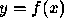example, let an approximate solution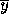satisfy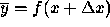. Then, assuming that f is twice differentiable,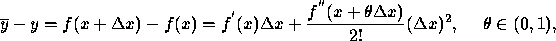and we can bound or estimate the right hand side. This expansion leads to the notion of condition number. Since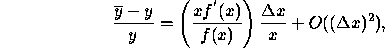the quantity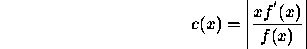measures, for small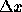, the relative change in the output for a given relative change in the input, and it is called the condition number of f. If x or f is a vector then the condition number is defined in a similar way using norms (we will cover them later in the course) and it measures the maximum relative change. In general, the forward error, backward error and the condition number satisfy the following rule: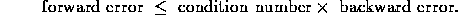The condition number of a problem is always greater than or equal to one. A problem is ill-conditioned if its condition number is high. It is ill-posed if its condition number is very high (say infinity).

Dinesh Manocha
Wed Jan 8 00:43:08 EST 1997